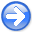A reversible transform for seismic data processingNext: Introduction Up: Reproducible Documents

### Abstract:

We use the nonstationary equivalent of the Fourier shift theorem to derive a general one-dimensional integral transform for the application and removal of certain seismic data processing steps. This transform comes from the observation that many seismic data processing steps can be viewed as nonstationary shifts. The continuous form of the transform is exactly reversible, and the discrete form provides a general framework for unitary and pseudounitary imaging operators. Any processing step which can be viewed as a nonstationary shift in any domain is a special case of this transform. Nonstationary shifts generally produce coordinate distortions between input and output domains, and those that preserve amplitudes do not conserve the energy of the input signal. The nonstationary frequency and time distortions and nonphysical energy changes inherent to such operations are predicted and quantified by this transform. Processing steps of this type are conventionally implemented using interpolation operators to map discrete data values between input and output coordinate frames. Although not explicitly derived to perform interpolation, the transform here assumes the Fourier basis to predict values of the input signal between sampling locations. We demonstrate how interpolants commonly used in seismic data processing and imaging approximate the proposed method. We find that our transform is equivalent to the conventional sinc-interpolant with no truncation. Once the transform is developed, we demonstrate its numerical implementation by matrix-vector multiplication. As an example, we use our transform to apply and remove normal moveout.

# A reversible transform for seismic data processing

William A. Burnettand Robert J. FergusonJohn A. and Katherine G. Jackson School of Geosciences, The University of Texas at Austin, University Station, Box X, Austin, TX 78713-8972.Department of Geoscience, University of Calgary, 2500 University Drive, N.W., Calgary, Canada T2N 1N4.A reversible transform for seismic data processingNext: Introduction Up: Reproducible Documents

2013-03-02